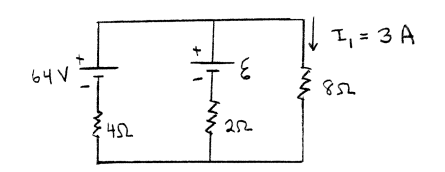# Problem: Consider the circuit shown in the sketch. The current in the 8.0 Ω resistor is   I1 = 3.0 A. What is the current through 4.0 Ω resistor?

###### FREE Expert Solution
98% (478 ratings)
###### Problem Details

Consider the circuit shown in the sketch. The current in the 8.0 Ω resistor is   I= 3.0 A. What is the current through 4.0 Ω resistor?